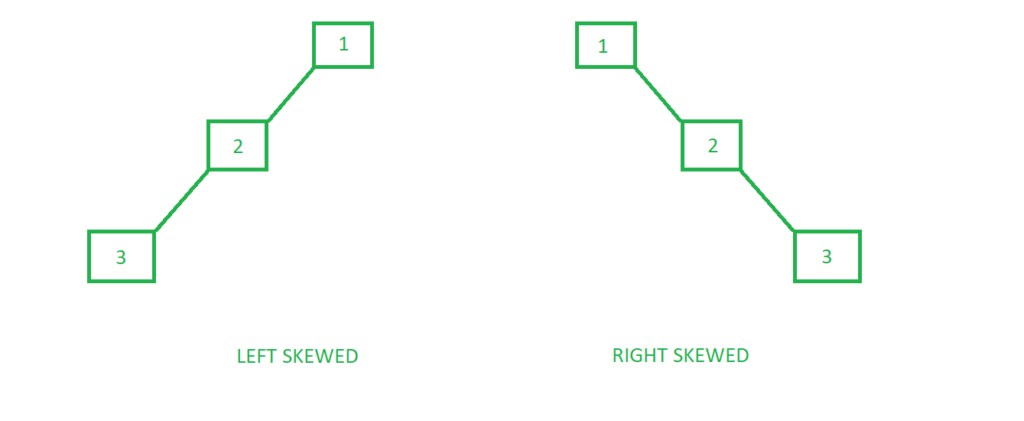GeeksforGeeks App
Open AppBrowser
Continue

A skewed binary tree is a type of binary tree in which all the nodes have only either one child or no child.

Types of Skewed Binary trees

There are 2 special types of skewed tree:1. Left Skewed Binary Tree:
These are those skewed binary trees in which all the nodes are having a left child or no child at all. It is a left side dominated tree. All the right children remain as null.

Below is an example of a left-skewed tree:

## C++

 `#include ``using` `namespace` `std;` `// A Tree node``struct` `Node {``    ``int` `key;``    ``struct` `Node *left, *right;``};` `// Utility function to create a new node``Node* newNode(``int` `key)``{``    ``Node* temp = ``new` `Node;``    ``temp->key = key;``    ``temp->left = temp->right = NULL;` `    ``return` `(temp);``}` `// Driver code``int` `main()``{``    ``/*``            ``1``           ``/``          ``2``         ``/``        ``3``    ``*/``    ``Node* root = newNode(1);``    ``root->left = newNode(2);``    ``root->left->left = newNode(3);` `    ``return` `0;``}`

## Java

 `// Java implementation of above approach``import` `java.util.*;` `class` `GFG``{``    ` `// A Tree node``static` `class` `Node``{``    ``int` `key;``     ``Node left, right;``};``  ` `// Utility function to create a new node``static` `Node newNode(``int` `key)``{``    ``Node temp = ``new` `Node();``    ``temp.key = key;``    ``temp.left = temp.right = ``null``;``  ` `    ``return` `(temp);``}``  ` `// Driver code``public` `static` `void` `main(String args[])``{``    ``/*``            ``1``           ``/``          ``2``         ``/``        ``3``    ``*/``    ``Node root = newNode(``1``);``    ``root.left = newNode(``2``);``    ``root.left.left = newNode(``3``);``}``}` `// This code is contributed by Arnab Kundu`

## Python3

 `# Python3 implementation of the above approach` `# Class that represents an individual``# node in a Binary Tree``class` `Node:``    ``def` `__init__(``self``, key):``        ` `        ``self``.left ``=` `None``        ``self``.right ``=` `None``        ``self``.val ``=` `key``        ` `# Driver code` `"""         1``           ``/``          ``2``         ``/``        ``3     """``root ``=` `Node(``1``)``root.left ``=` `Node(``2``)``root.left.left ``=` `Node(``2``)` `# This code is contributed by dhruvsantoshwar`

## C#

 `// C# implementation of above approach``using` `System;`` ` `class` `GFG``{``         ` `    ``// A Tree node``     ``public` `class` `Node``    ``{``         ``public` `int` `key;``         ``public` `Node left, right;``    ``};``         ` `    ``// Utility function to create a new node``     ``static` `Node newNode(``int` `key)``    ``{``        ``Node temp = ``new` `Node();``        ``temp.key = key;``        ``temp.left = temp.right = ``null``;``         ` `        ``return` `(temp);``    ``}``         ` `    ``// Driver code``    ``public` `static` `void` `Main()``    ``{``        ``/*``                ``1``            ``/``            ``2``            ``/``            ``3``        ``*/``        ``Node root = newNode(1);``        ``root.left = newNode(2);``        ``root.left.left = newNode(3);``    ``}``}` `// This code is contributed by AnkitRai01`

## Javascript

 ``

2. Right Skewed Binary Tree:
These are those skewed binary trees in which all the nodes are having a right child or no child at all. It is a right side dominated tree. All the left children remain as null.

Below is an example of a right-skewed tree:

## C++

 `#include ``using` `namespace` `std;` `// A Tree node``struct` `Node {``    ``int` `key;``    ``struct` `Node *left, *right;``};` `// Utility function to create a new node``Node* newNode(``int` `key)``{``    ``Node* temp = ``new` `Node;``    ``temp->key = key;``    ``temp->left = temp->right = NULL;` `    ``return` `(temp);``}` `// Driver code``int` `main()``{``    ``/*``        ``1``         ``\``          ``2``           ``\``            ``3``    ``*/``    ``Node* root = newNode(1);``    ``root->right = newNode(2);``    ``root->right->right = newNode(3);` `    ``return` `0;``}`

## Java

 `// Java implementation of above approach``import` `java.util.*;``class` `GFG``{``    ` `// A Tree node``static` `class` `Node``{``    ``int` `key;``    ``Node left, right;``};``  ` `// Utility function to create a new node``static` `Node newNode(``int` `key)``{``    ``Node temp = ``new` `Node();``    ``temp.key = key;``    ``temp.left = temp.right = ``null``;``  ` `    ``return` `(temp);``}``  ` `// Driver code``public` `static` `void` `main(String args[])``{``    ``/*``       ``1``        ``\``         ``2``          ``\``           ``3``    ``*/``    ``Node root = newNode(``1``);``    ``root.right = newNode(``2``);``    ``root.right.right = newNode(``3``);``}``}` `// This code is contributed by Arnab Kundu`

## Python3

 `# Python3 implementation of the above approach` `# A Tree node``class` `Node:``    ` `    ``def` `__init__(``self``, key):``        ` `        ``self``.left ``=` `None``        ``self``.right ``=` `None``        ``self``.val ``=` `key``        ` `# Driver code``"""        ``        ``1``         ``\``          ``2``           ``\``            ``3``                 ``"""``root ``=` `Node(``1``)``root.right ``=` `Node(``2``)``root.right.right ``=` `Node(``3``)` `# This code is contributed by shivanisinghss2110`

## C#

 `// C# implementation of above approach``using` `System;`` ` `class` `GFG``{``      ` `// A Tree node``public` `class` `Node``{``    ``public` `int` `key;``    ``public` `Node left, right;``};``    ` `// Utility function to create a new node``static` `Node newNode(``int` `key)``{``    ``Node temp = ``new` `Node();``    ``temp.key = key;``    ``temp.left = temp.right = ``null``;``    ` `    ``return` `(temp);``}``    ` `// Driver code``public` `static` `void` `Main(String []args)``{``    ``/*``       ``1``        ``\``         ``2``          ``\``           ``3``    ``*/``    ``Node root = newNode(1);``    ``root.right = newNode(2);``    ``root.right.right = newNode(3);``}``}`` ` `// This code is contributed by PrinciRaj1992`

## Javascript

 ``

My Personal Notes arrow_drop_up